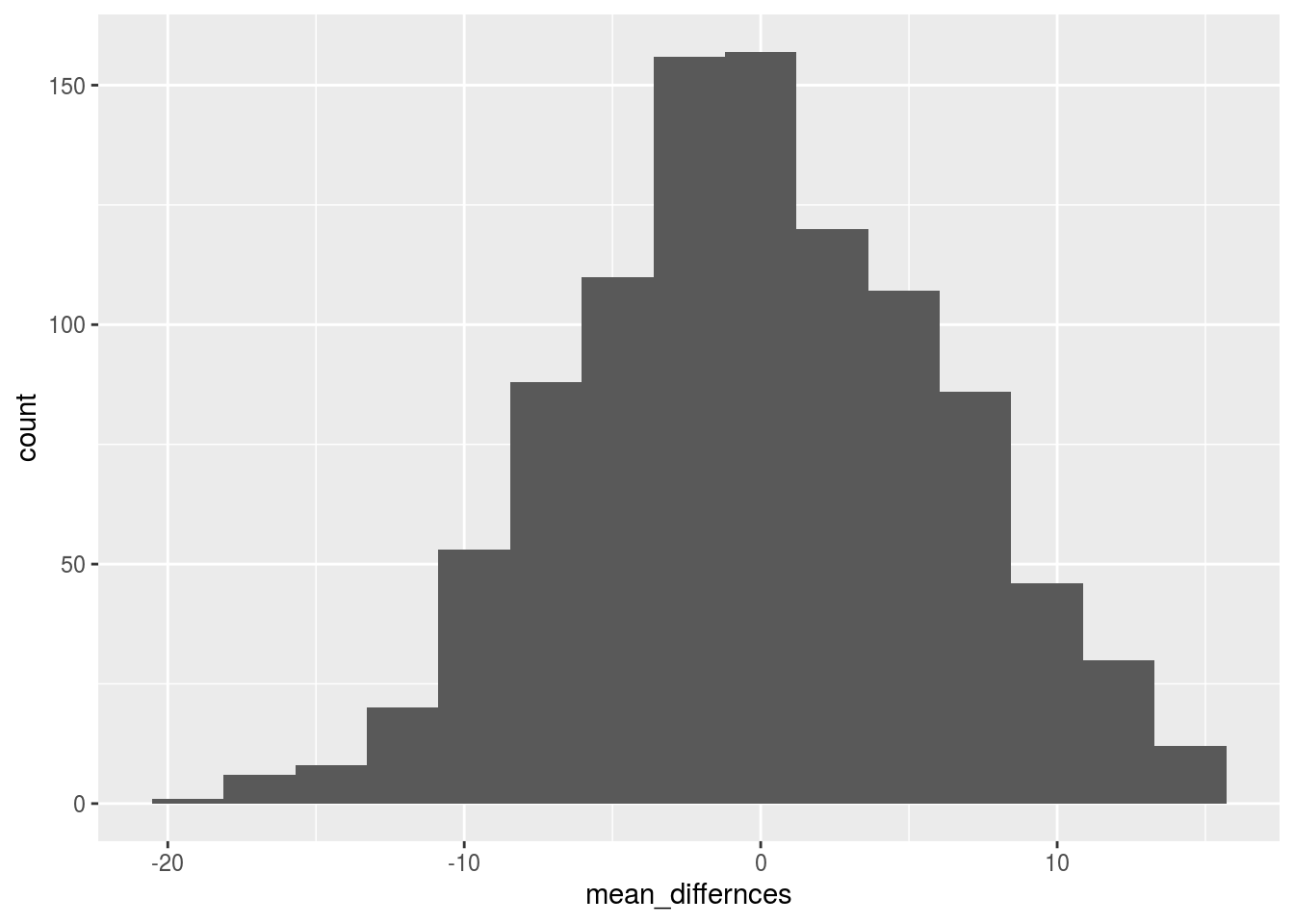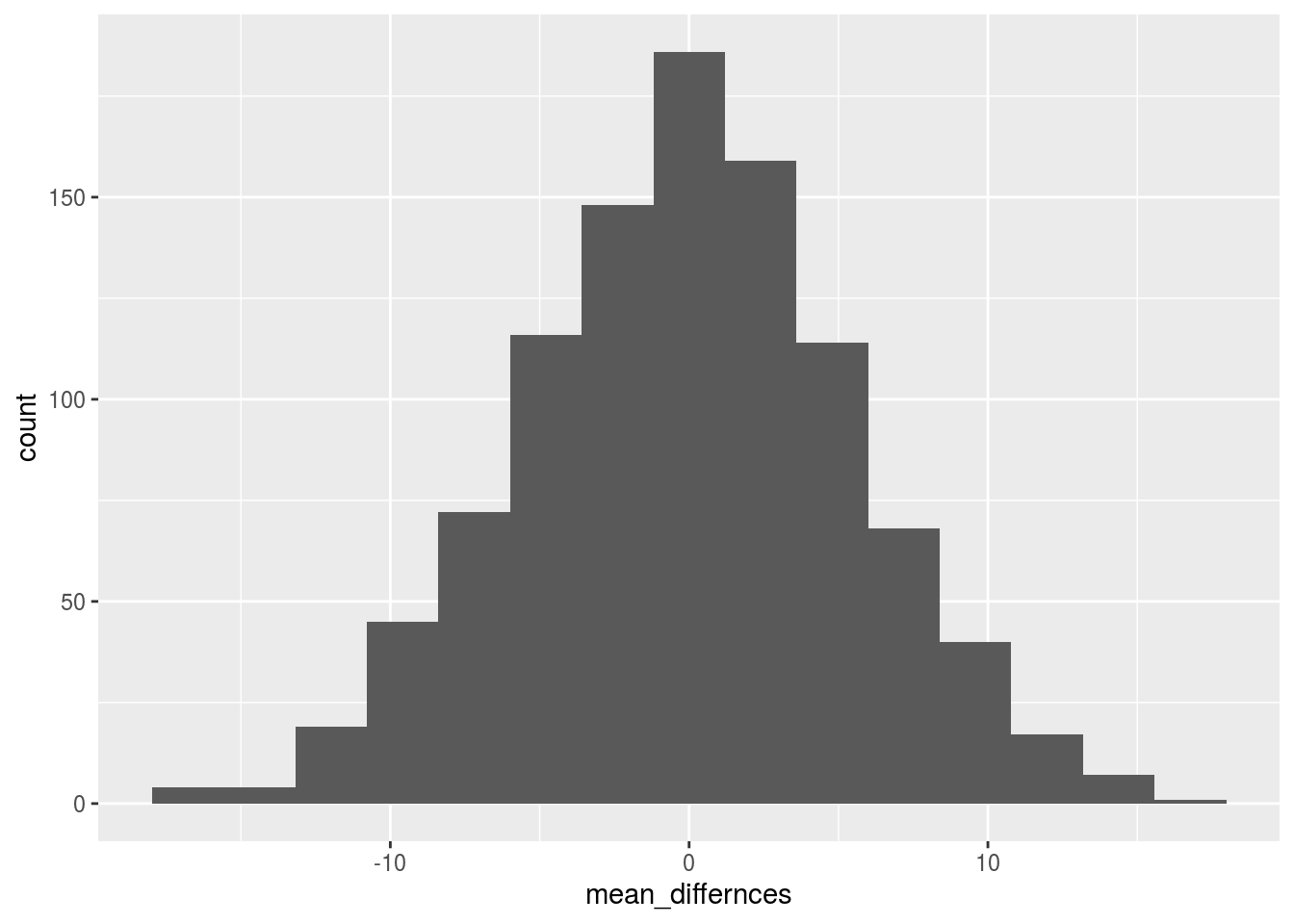Now that we have covered the basics of the Central Limit Theorem, today we move one step ahead and discuss hypothesis testing, the gold-standard practice in science.

Hypothesis testing is very much like the saying that “every person is innocent until proven guilty beyond a reasonable doubt”. Similarly, in hypothesis testing, we consider each estimate (e.g., mean, the difference between groups) as a random estimate (i.e., innocent) until we prove that chance can’t produce this estimate. So the presumption of innocence is the presumption of chance in the world of statistics.

What is reasonable doubt? Let’s take a more practical example. You found a magic pill that improves memory. To test its effectiveness, you need to run an experiment. So you sampled random students from Brooklyn College and assigned them to two groups: the control group and the experimental group. For the experimental group, you gave them the magic pill just before they study for their exam. For the control group, you gave them a placebo pill that does nothing. After their memory test, you now have two sets of grades: those from your control group and those from the experimental group. If you take the mean of the two groups and subtract the control mean from the experimental group mean, you found the average difference is 6% (meaning, the grades of the experimental group were, on average, 6% better than the grades of the control group).

In hypothesis testing, you first start with defining the alternative hypothesis which is the magic pill improves students’ memory (and hence their grades are better). The null hypothesis is that the magic pill does not improve students’ memory. The basic concept is that we can’t prove that the pill actually improves students’ memory because you can’t calculate the probability of scoring 6% better if you take the pill. However, we can calculate the probability of achieving 6% better by chance, and then decide for ourselves if this probability is “beyond the reasonable doubt” or, in a more statistical context, if this probability is less than our alpha criterion (5%). If it is less than alpha, then we reject the null hypothesis and conclude that this 6% is very unlikely to be produced by chance. However, if the probability is bigger than alpha, then we say that we failed to reject the null hypothesis. Be Careful: this does not prove that the null hypothesis is true! If a person is proved to be innocent beyond a reasonable doubt, that does not mean this person did not commit the crime.

Let’s now talk simulations. We will simulate two groups with the same parameters (mean and std) from a normal distribution (or any distribution you like), then calculate the mean difference between the two groups, and finally visualize the distribution of those differences. I will repeat this process 100 times

mean_differnces <- c()
sample_size <- 20
n_exps <- 1000
pop_mean <- 70
pop_sd   <- 20
for (i in 1:n_exps){
group_experimental <- rnorm(n=sample_size, mean=pop_mean, sd=pop_sd)
group_control      <- rnorm(n=sample_size, mean=pop_mean, sd=pop_sd)
mean_experimental <- mean(group_experimental)
mean_control      <- mean(group_control)
mean_diff         <- mean_experimental- mean_control
mean_differnces[i] <- mean_diff
}

Now, since both distributions are coming from the same mean and standard deviation, we know that any reported difference is only possible due to chance (random sampling). Let’s investigate those mean differences:

range(mean_differnces)
##  -18.20509  15.61411

Ops! we can actually observe large differences, even though those differences are purely due to random sampling. Let’s make a histogram

library(ggplot2)
ggplot(data.frame(mean_differnces), aes(x=mean_differnces)) + geom_histogram(bins = 15)We see that we have a few extreme values, but most values are actually closer to zero. This is the range of differences that could be obtained if NO manipulation took place. In another world, this is the null distribution of differences. According to the CLT, we know that this distribution should approximate a normal distribution if we increased the sample size of each group.

Since we know how to calculate the probabilities from a normal distribution, that means we can now calculate the probability of observing any difference by chance. Let’s calculate the probability of observing a difference of 6% or higher, but using the samples we have

mean(mean_differnces > 6 | mean_differnces < -6)
##  0.353

And using pnorm that gives us the true mathematical probability if we sampled an infinite number of subjects in both groups:

pnorm(q=6, mean=mean(mean_differnces), sd = sd(mean_differnces), lower.tail = FALSE) +
pnorm(q=-6, mean=mean(mean_differnces), sd = sd(mean_differnces), lower.tail = TRUE)
##  0.333289

This is the role of chance in this experiment. Is that role small enough for you beyond the reasonable doubt to reject the null hypothesis and claim a victory? Well, we usually set alpha to 5% or lower, so that won’t be considered significant by scientific standards.

Does that make your magic pill effectless? Not at all. Let’s repeat the same simulation but with a much larger sample (N=200 this time):

mean_differnces <- c()
sample_size <- 200
n_exps <- 1000
pop_mean <- 70
pop_sd   <- 20
for (i in 1:n_exps){
group_experimental <- rnorm(n=sample_size, mean=pop_mean, sd=pop_sd)
group_control      <- rnorm(n=sample_size, mean=pop_mean, sd=pop_sd)
mean_experimental <- mean(group_experimental)
mean_control      <- mean(group_control)
mean_diff         <- mean_experimental- mean_control
mean_differnces[i] <- mean_diff
}

And now we calculate the probability of observing 6% by chance:

mean(mean_differnces > 6 | mean_differnces < -6)
##  0.001

The probability now is so small that it can actually be your chance to enter the billionaire club with the magic pill. But 6% is actually not that big! A small probability of chance does not mean the magnitude of the effect is big or meaningful in any way.

# The Randomization Test

Until now, we have assumed that the difference scores are normally distributed. And the difference scores are normally distributed because we are sampling both groups from the same normal distributions. However, that may not be the case with all the things we are interested in. What should we do to calculate the probability of chance given any data (or any distribution)? Try the randomization test. It is a non-parametric method that we’ll begin to use more often to make inferential statements. Basically, we no longer require the real-world to behave according to our math but that means we’ll reply on simulations to make inferential statements. Let’s explain how the randomization test works in the context of hypothesis testing.

You first have two sets of scores from your experiment, one for the control group and the other from the experimental group. You take the difference between the two, and you want to calculate the probability of observing this difference by chance. We’ll first calculate the observed difference. Then, to make the null distribution, we repeatedly shuffle group assignments and calculate the difference. After we do that multiple times, we can now use this distribution of differences (under shuffled group assignments) as our null distribution and use it to calculate the probability.

Let’s see how to do that:

sample_size <- 20
n_exps <- 1000
pop_mean <- 70
pop_sd   <- 20
group_experimental <- rnorm(n=sample_size, mean=pop_mean, sd=pop_sd)
group_control      <- rnorm(n=sample_size, mean=pop_mean, sd=pop_sd)
group_score_both <- c(group_experimental, group_control)
group_assignments <- rep(c("A","B"), each=20)

Let’s see what both group_scores_both and group_assignments look like:

head(group_score_both)
##  60.40451 87.56891 71.95822 42.40252 75.02995 29.60500
head(group_assignments)
##  "A" "A" "A" "A" "A" "A"

Basically, the first score is from group “A”, second from group “A” and etc.

let’s review what sampling without replacement does:

sample(group_assignments, replace=FALSE)
##   "A" "B" "A" "A" "A" "B" "A" "B" "B" "B" "A" "B" "B" "A" "B" "B" "B"
##  "B" "A" "A" "B" "A" "A" "A" "B" "A" "B" "B" "B" "B" "A" "A" "B" "A"
##  "B" "A" "A" "A" "A" "B"

As you can see, sampling without replacement will just shuffle the letters all around the place and break their order.

Now, we will perform the randomization test. Basically, shuffle group assignments by random, and then calculate the difference.

mean_differnces <- c()
# Note, we already have a set of scores for both groups
for (i in 1:n_exps){
shuffled_assignments <- sample(group_assignments, replace=FALSE)   # without replacement
group_experimental_shuffled <- group_score_both[shuffled_assignments=='A']
group_control_shuffled <- group_score_both[shuffled_assignments=='B']
mean_experimental <- mean(group_experimental_shuffled)
mean_control      <- mean(group_control_shuffled)

mean_diff         <- mean_experimental- mean_control
mean_differnces[i] <- mean_diff
}

Now we can make a distribution of those differences (using the absolute value, because we are doing a two-tailed or non-directional test).

library(ggplot2)
ggplot(data.frame(mean_differnces), aes(x=mean_differnces)) + geom_histogram(bins = 15)Those differences are examples of what you would expect if there were no effect. If the observed difference looks like all those differences, then we can conclude that chance might have produced those effects. However, if it looks more extreme, then we can conclude that it is very unlikely to be produced by chance.

We can assume that our alpha criterion is 5%, we can now calculate the critical value (the least difference that would be accepted):

quantile(mean_differnces, c(0.025, 0.975)) # calcuating 5% divided by two tails: 2.5% each
##      2.5%     97.5%
## -11.05458  10.76974

Now, any observed difference that is between the range of those two numbers would be a failure to reject the null hypothesis. All the observed differences that are outside that range would only occur 5% of the time and hence we can reject the null hypothesis.

Having observed 6%, we can simply calculate the probability of that difference:

mean(mean_differnces < -6 | mean_differnces > 6)
##  0.275

Which is much higher than our alpha (and inside the range).

# Exercises

Make a simulation of two groups (N=10 per group) drawn from the same population with the following parameters: mean=40 and sd=10.

• Use the CLT to find the probability of observing a difference of 4 or more between the two groups (either positive or negative), by chance.

• Calculate the same probability using the randomization test.

• Given the similarity of the two probabilities, what is the biggest advantage of using the randomization test?

• While keeping everything else fixed, what happens to those probabilities if we increase the number of subjects (N) from 10 to 1000 – and why? (no need to provide the code, just write what happens and the reason you think behind this effect)